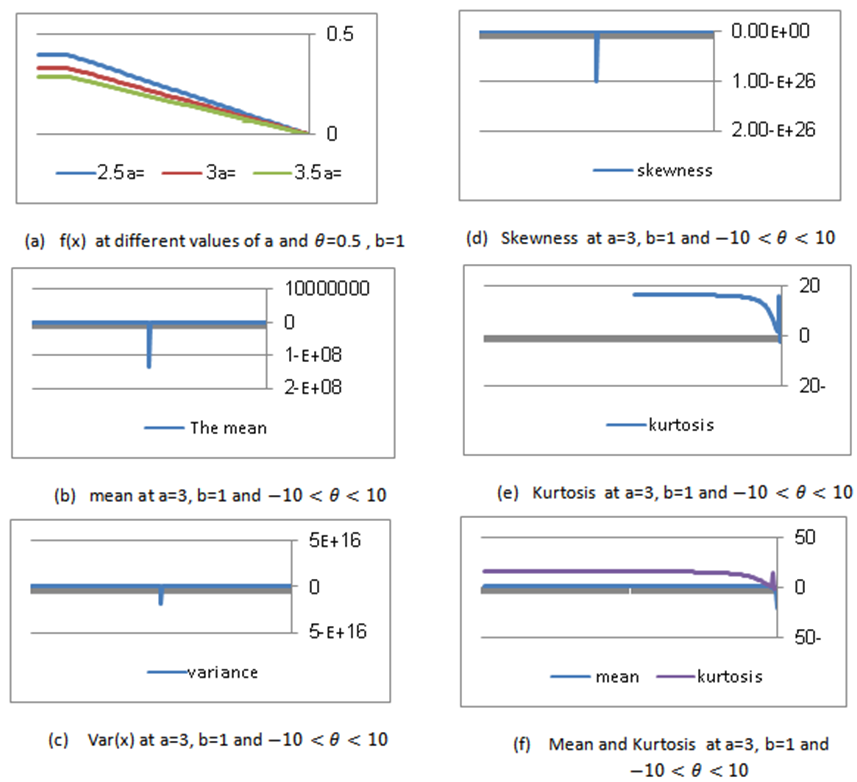## Figure 1. (a) the shape of f(x) at different values of a and =0.5 , b=1; (b) mean at a=3, b=1 and; (c) Var(x) at a=3, b=1 and; (d) Skewness at a=3, b=1 and; (e) Kurtosis at a=3, b=1 and; (f) Mean and Kurtosis at a=3, b=1 and#### Salah H Abid

American Journal of Applied Mathematics and Statistics. 2015, 3(4), 164-167 doi:10.12691/ajams-3-4-6